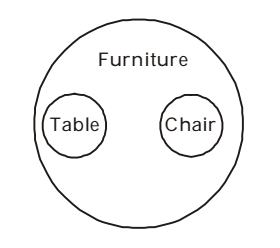## Logical Venn Diagram

#### Logical Venn Diagram

1. Which of the following diagrams represents the relationship of Profit, Dividend, Bonus?

1. From above given question , we can say that
Bonus and Dividend are different from each other but both these are parts of profit.

##### Correct Option: B

From above given question , we can say that
Bonus and Dividend are different from each other but both these are parts of profit.
From above it is clear that option ( B ) represents the best relationship between Profit, Dividend and Bonus . As shown in given venn - diagram .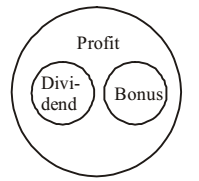1. If each circle represents a class of objects/ideas, written below then find out the answer figure which illustrates better the relationship among them: Cats, Rats, Animals

1. According to above given question , we know that
Cats are different from rats.
But both are animals.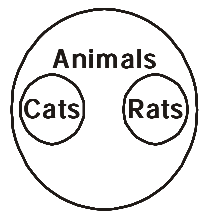##### Correct Option: D

According to above given question , we know that
Cats are different from rats.
But both are animals.
From above it is clear that option ( D ) represents the best relationship between Cats, Rats and Animals . As shown in given venn - diagram .1. Some of the Cricket players are Tennis players, Some Tennis players are Hockey players, no Cricket player is a Hockey player. Which one of the following diagrams represents the above statements?

1. According to above all given informations in question , we can say that
Diagram ( B ) represents the best relationship between Cricket players , Tennis players and Hockey players .

##### Correct Option: B

According to above all given informations in question , we can say that
Diagram ( B ) represents the best relationship between Cricket players , Tennis players and Hockey players .As shown in given venn - diagram .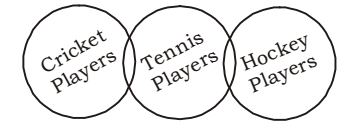1. Which one of the following diagrams best depicts the relationship among Pigeons, Birds and Dogs?

1. On the basis of above given questions , we find the best relationship between them .
Pigeons come under the class Birds.
But dog is different (mammal).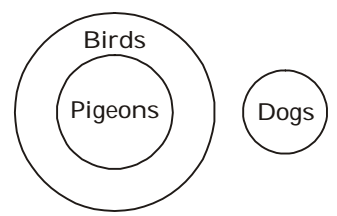##### Correct Option: A

On the basis of above given questions , we find the best relationship between them .
Pigeons come under the class Birds.
But dog is different (mammal).
It is clear that option ( A ) represents the best relationship between Pigeons, Birds and Dogs . As shown in given venn - diagram .1. Which one of the following figures represents the relationship among Tables, Chairs, Furniture?

1. As per the above given question , we can say that
Chair and Table are two types of Furniture and table and chair are different from each other.

##### Correct Option: D

As per the above given question , we can say that
Chair and Table are two types of Furniture and table and chair are different from each other.
Therefore , option ( D ) gives the best relationship between Tables, Chairs and Furniture.